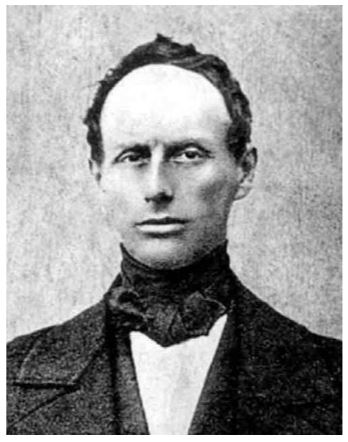$$\require{cancel}$$

# 9.7: The Doppler Effect

The Doppler effect is a physical phenomenon that most people have experienced many times: when a moving source of sound (say an ambulance, or more exactly its siren) is approaching you, its pitch sounds noticeably higher then after it passed you by and is moving away. The effect is due to the fact that the observed wavelength (and therefore frequency / pitch) of sound corresponds to the distance between two points of equal phase (i.e., two sequential wavefronts). Ultimately, the Doppler effect thus originates in a change in reference frame (the same frames we encountered in Section 4.3): what you hear is indeed different from what the ambulance’s driver hears. The latter is easy: the driver isn’t moving with respect to the siren, so (s)he simply hears it at whatever frequency it is emitting. For the stationary observer however, the ambulance moves between emitting the first and second wave crest, and so their distance (and hence the observed wavelength / frequency) changes, see Figure 9.7.2.

Christian doppler

Christian Doppler (1803-1853) was an Austrian physicist. Doppler was a professor of physics at Prague where he developed the notion that the observed frequency of a wave depends on the relative speed of the source and the observer, now known as the Doppler effect. Doppler used this principle to explain the observed colors of binary stars. The principle was developed independently by French physicist Armand Fizeau (1819-1896), and is therefore sometimes referred to as the Doppler-Fizeau effect. In 1847, Doppler moved to Selmecbánya in Hungary, but was forced to leave again soon afterwards due to political unrest in 1848, moving to the university of Vienna. During a visit to Venice in 1853, Doppler died of pulmonary disease, aged only 49.Figure $$\PageIndex{1}$$: Christian Doppler .Figure $$\PageIndex{2}$$: Doppler effect. The source emits waves with a fixed time interval $$\Delta t$$ between successive wavefronts. (a) For observers that are stationary with respect to the source, the distance between wavefronts (in red) is fixed, so they measure the same wavelength as the one emitted by the source. (b) If the source is moving with respect to the observers (here to the right), the observers measure a different distance between arriving wavefronts - compressed (so shorter wavelength / higher frequency) if the source is approaching (green dot), expanded if the source is receding (purple dot).

ernst mach

Ernst Mach (1838-1916) was an Austrian physicist. Mach was a professor of mathematics and later physics at Graz, Prague and Vienna. His experimental work focused on the properties of waves, especially in light, as well as on the Doppler effect in both light and sound. In 1888, Mach used photography to capture the shock waves created by a supersonic bullet. In addition to physics, Mach was highly interested in philosophy, holding the position that only sensations are real. Consequently, Mach refused to accept that atoms are real, as they could not be observed directly at the time; it was Einstein’s 1905 work on Brownian motion that eventually proved him wrong. The ratio of an object’s speed to the speed of sound is now known as the Mach number in his honor.Figure $$\PageIndex{3}$$: Left: Mach’s picture of the shockwave of a supersonic bullet . Right: Ernst Mach (1902) .

Calculating the shift in wavelength is straightforward. Let us call the speed of sound $$v$$ and the speed of the source $$u$$. The time interval between two wavefronts, as emitted by the source, is $$\Delta t$$. In this time interval, the first wavefronts travels a distance $$\Delta s=v \Delta t$$, while the source travels a distance $$\Delta x=u \Delta t$$. For the observer to which the source is approaching, the actual distance between two emitted wavefronts is thus $$\Delta x^{\prime}=\Delta s-\Delta x=(v-u) \Delta t$$. The actual distance between the wavefronts is the observed wavelength, $$\lambda _{obs}$$, while the emitted wavelength is $$\lambda=\Delta s=v \Delta t$$, so the two are related through

$\lambda_{\mathrm{obs}}=\frac{v-u}{v} \lambda \label{9.19}$

For a source that is moving away, we simply flip the sign of $$u$$; naturally for a stationary source we have $$\lambda _{obs} = \lambda$$. Note that we could also consider a stationary source and a moving observer: the effect would be exactly the same, where in Equation \ref{9.19} we define motion towards the source to be the positive direction.

The Doppler effect is usually expressed in terms of frequency instead of wavelength, but that is a trivial step from Equation \ref{9.19}, as $$f_{\mathrm{obs}}=v / \lambda_{\mathrm{obs}}$$ and $$f=v / \lambda$$, which gives:

$f_{\mathrm{obs}}=\frac{v}{v-u} f$

Although we discussed the Doppler effect here in the context of sound waves, it occurs for any kind of waves - most notably also light. We will encounter it again when we discuss waves in special relativity (where speeds become comparable to that of light) in Section 15.3. Note that Equation \ref{9.19} predicts that the wavelength is zero if the speed of the source equals the speed of the emitted waves. This case is illustrated in Figure 9.7.4a, which shows that the wavefronts pile up. For a source moving faster than the wave speed (Figure 9.7.4b), the waves follow the source, creating a conical shock wave, with opening angle given by

$\sin \theta=\frac{v T}{u T}=\frac{v}{u}$

Both the bow wave of a boat and the sonic boom of a supersonic jet are examples of shock waves.Figure $$\PageIndex{4}$$: Shock waves. Like in Figure 9.7.2, the source emits wavefronts with a fixed time interval $$\Delta t$$. (a) If the source is moving at the same speed as the emitted wave, the wavefronts all collide, creating a shock wave. (b) For a source moving faster than the speed of the waves, the waves all travel behind the source, creating a conical (shock) wave front (as you may have heard after seeing a jet fighter pass overhead). The blue dot indicates the current position of the source, the green dot that one period ago, the red dot two periods ago.